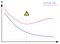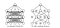# Regularization Techniques in Machine Learning

Generally, the output of a model can be affected by multiple features. When the number of features increases, the model becomes complicated.

An overfitting model tends to take all the features into consideration, even though some of them have a very limited effect on the final output. Or even worse, some of them are noises that are meaningless to the output.

We need to limit the effect of these useless features. However, we do not always know which features are useless, so we try to limit them all by minimizing the cost function of our model. To do so we have regularization techniques.

It is assumed that you are familiar with overfitting and underfitting. If not, check out the following article Investigating Underfitting and Overfitting

Regularization helps to overcome overfitting while developing machine learning models. These techniques intend to reduce the risk of overfitting without increasing the bias significantly.

There are several ways to regularize a model. In this article, we will discuss in detail three general regularization methods:

• Early Stopping
• Weight decay
• Dropout

Early Stopping is used to avoid the phenomenon of “ you are learning speed slow-down ”. This issue means that the accuracy of algorithms stops improving after some point, or even gets worse because of noise-learning. If the model continues learning after the point, the validation error will increase while the training error will continue decreasing.Early stopping

Also, If we stop learning before the point, it’s under-fitting. If we stop after the point, we get over-fitting. So the aim is to find the exact point to stop training. In this way, we will get a perfect fit between under-fitting and overfitting.

Practically, to find out the point to stop learning, the obvious way is to keep track of accuracy on the validation data as our model trains. In another word, we compute the accuracy at the end of each epoch and stop training when the accuracy of the validation set stops improving.

We use a validation set to figure out a perfect set of values for the hyper-parameters, and later use the test set to do the final evaluation of accuracy. In this way, a higher generalization model can be guaranteed.

This strategy ensures that, at each step of an iterative algorithm, bias is reduced but the variance is increased. So finally, the variance of the estimator will not be too high. Besides, it has a lower computational complexity.

However, there is a problem, in some situations where validation accuracy stops improving is not a necessary sign of overfitting. It might be that the accuracy of both the validation set and the training data stops improving at the same time.

A very common regularization strategy is to add a weight decay term to the loss function. This weight decay term puts a constraint on the model complexity by penalizing large weights.

It is assumed that you are familiar with linear regression. If not, check out the following article Linear Regression in a Nutshell

So as you know in linear regression, we consider the least square as a loss function and try to minimize it using the ordinary least square method to obtain values of coefficients (β₀, β₁, … βₙ).

The loss function is given as

But in linear regression with regularization, we add a weight decay term to this loss function. And while minimizing regularized loss function, our algorithm tries to decrease both the original loss function and the weight decay term, expressing a preference towards smaller weights.

There are three different methods in weight decay

• L1 regularization or Lasso regression
• L2 regularization or Ridge regression
• Elastic Net regression

The L1 regularization modifies the overfitted or under-fitted models by adding the penalty equivalent to the sum of the absolute values of coefficients.

The loss function is given as

alpha (α) can be any value from 0 to positive infinity

A key property of L1 regularization is that it leads to sparser weights. In other words, it drives less important weights to zero, therefore acting as a natural feature selector. In this way, we can get a simpler model which is easier to interpret.

The reason behind that is that L1 regularization penalizes smaller weights less than the larger weights since it tries to minimize the squared magnitude of the weights and it removes some features from our model and keeps only valuable features.

However, at the same time, we lost some useful features which have a lower influence on the final output.

Ridge regression or L2 regularization modifies the overfitted or under-fitted models by adding the penalty equivalent to the sum of the squares of the magnitude of coefficients.

The loss function is given as

Compared to L1 regularization, this approach makes the networks prefer to learn features with small weights. Instead of rejecting those less valuable features, our algorithm gives them lower weights. So, we get as much information as possible. Large weights can only be given to the features that considerably improve the initial cost function.

Difference between Ridge and Lasso regression

• Ridge regression squares the weights and Lasso regression takes the absolute value.
• Ridge Regression can only shrink the weights asymptotically close to 0 while Lasso Regression can shrink the weights all the way to 0 which helps to exclude useless variables from the model and makes the model less complex.

Just like lasso and ridge regression, Elastic-net regression starts with least-squares then combines the lasso regression penalty with the ridge regression penalty.

Altogether, Elastic Net regression combines the strengths of lasso and ridge regression.

The loss function is given as

The hybrid Elastic-Net Regression is especially good at dealing with situations when there are correlations between parameters. This is because, on its own, Lasso Regression tends to pick just one of the correlated terms and eliminates the others whereas Ridge Regression tends to shrink all of the parameters for the correlated variables together.

By combining Lasso and Ridge Regression, Elastic-Net Regression groups and shrinks the parameters associated with the correlated variables and leaves them in the equation or removes them all at once.

It is assumed that you are familiar with neural networks. If not, check out the following article A Study of Artificial Neural Networks (ANN)

Dropout is a popular and effective technique against overfitting in neural networks. The initial idea of dropout is to randomly drop units and relevant connections from the neural networks during training. This prevents units from co-adapting too much.Standard Neural Network vs Neural Network with dropouts

Basically, the training algorithm uses a random subset of the network at every iteration. This approach encourages neurons to learn useful features on their own without relying on other neurons.

Once the model is trained the entire network is used for inference. The outputs of the neurons are scaled to make sure that the overall magnitude of the neuron outputs doesn’t change due to the changes number of active neurons during training and test.

Dropout reduces significantly the number of computations. This makes it an effective choice for big or complicated networks which need lots of training data.

The difference is that in dropout, the training algorithm doesn’t train disjoint models. Instead, a random sub-network is selected at every step. These sub-networks share parameters as they all come from the same network, but with a different set of units masked. In a way, Dropout can be considered a type of ensemble method that trains nearly as many models as the number of steps, where the models share parameters.

To prevent overfitting, we usually have two options: using methods that limit the effective capacity of the model or getting more training data. We discussed the methods to limit the effective capacity of the model in this article.

We can make use of L1 regularization if the model contains a lot of useless variables, L2 regularization when most of the variables in the model are useful, and Elastic Net regression if there are tons of features to be trained. Early stopping can be used to find the number of epochs for which we can train our model without overfitting it. In the case of neural networks, dropout layers can be used to decrease network complexity.

Thanks for reading this article! Leave a comment below if you have any questions. Be sure to follow @ArunAddagatla, to get notified regarding the latest articles on Data Science and Deep Learning.

You can connect with me on LinkedIn, Github, Kaggle, or by visiting Medium.com.

I am a Third-year Computer Engineering undergraduate student with an interest in Data Science, Deep Learning, and Computer Networking.

## More from Arun Addagatla

I am a Third-year Computer Engineering undergraduate student with an interest in Data Science, Deep Learning, and Computer Networking.